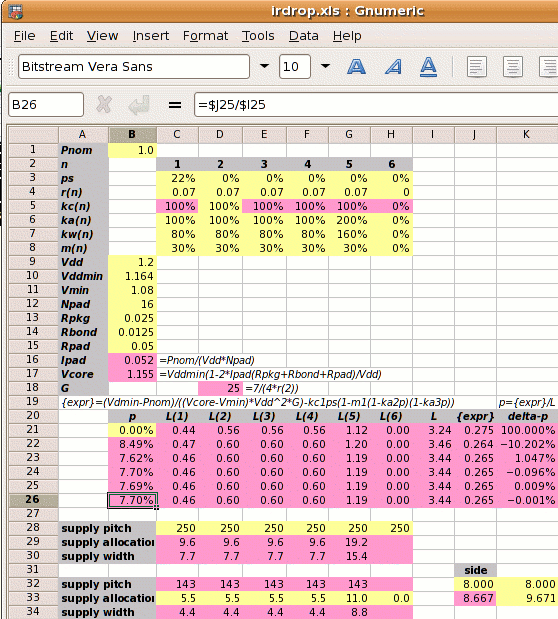Step 1: Calculate Ipad and Vcore:

1⁄(1.2×16) = 0.052A
Vcore =
1.164×(1−2×0.052×(0.025+0.0125+0.05)⁄1.2
1.155V

Step 2: Calculate the reference power supply conductance G:

G =
 7 4×r2

7 ⁄ (4 × 0.07) =  25 mhos

Step 3 is to set out the values of kan, kwn, kcn and mn for each metal layer, and use these to calculate the value of L.

metal layer 1 2 3 4 5 6
kan 100% 100% 100% 100% 200%   0%
power metal allocated coefficient
kwn  80%  80%  80%  80%  80%   0%
power metal used coefficient
kcn 100% 100% 100% 100% 100%   0%¹
conductivity coefficient
mn  30%  30%  30%  30%  30%   0%
core area blocked
¹no M6 layer

The value of L depends on p which we don't know. We iterate to the solution and use p=0 for the first estimate.

 L = kw1kc1(1-ps)(1-m1(1-ka2p)(1-ka3p))+ kw2kc2(1-m2(1-ka2p)(1-ka3p))+ kw3kc3(1-m3(1-ka2p)(1-ka3p))+ kw4kc4(1-m4(1-ka2p)(1-ka3p))+ kw5kc5(1-m5(1-ka2p)(1-ka3p)) = ( 0.44 + 0.56 + 0.56 + 0.56 + 1.12 ) = 3.24

Step 4: Calculate the power strap allocation percentage p. The solution must be iterated, and the calculation below shows the first iteration.

 m1′ = m1×(1-ka2p)(1-ka3p)
p =
 { Vddmin×Pnom −kc1×ps(1-m1′) } × 1 (Vcore−Vmin)×Vdd2×G L
 { 1.164×1 −1×0.22×(1-0.3) } × 1 (1.155−1.08)×1.22×25 3.24
(0.430−0.156)×0.309 = 8.49%

As shown on the right, a spreadsheet can be used to iterate to the answer of p=7.70%.

Step 5: Calculate the new core size. If the initial core size estimate without power straps is x, then with power straps the core size becomes x

 x′ = x = x = x = x+8.34% √(((1-ka2p)(1-ka3p)) √0.92302 0.9230

The value 8.34% is called the IR Drop Adder.

The width of the power straps is set by the pitch, strap allocation or width chosen by the user and the value of p just calculated. An example is shown in the table below, where we set the supply strap allocation to 5.5µm and compare it to the old solution.

p allocation   pitch   core side
met‑1‑4 met‑5
old 17.27% 5.5µm 5.5µm  64µm 9.671mm
new  7.70% 5.5µm 11µm 143µm 8.667mm

Design Attribute Value
Pnom core power consumption 1W
ps fraction of metal-1 in the standard cells used for power supplies 22% (for vsclib)
rn resistivity of metal layer n in ohms per square 0.07Ω per sq.
kan
 user defined   ratio of metal layer n allocated to power metal-2 allocated to power
100%
kwn
 user defined   ratio of metal layer n used for power metal-2 allocated to power
80%
mn percentage of metal layer n blocked to power straps 30%
Vdd the nominal supply voltage 1.2V
Vddmin the minimum supply voltage, 3% less than nominal 1.164V
Vmin the desired voltage at the centre of the die, 10% less than the nominal 1.08V
Rpkg the resistance of the package leadframe 25mΩ
Rbond the resistance of the bond wire 12.5mΩ¹
¹double bond halves bond wire resistance; ²two supply pads halves pad resistance

kcn =
 r2 rnThe new solution has allowed the vertical power strap pitch to go up from one every 64µm to one every 143µm. The core side is 1004µm less and the core area is 20% less than the first solution, due to double bonding of supply pads, tighter Vddmin spec and wider metal-5 straps.